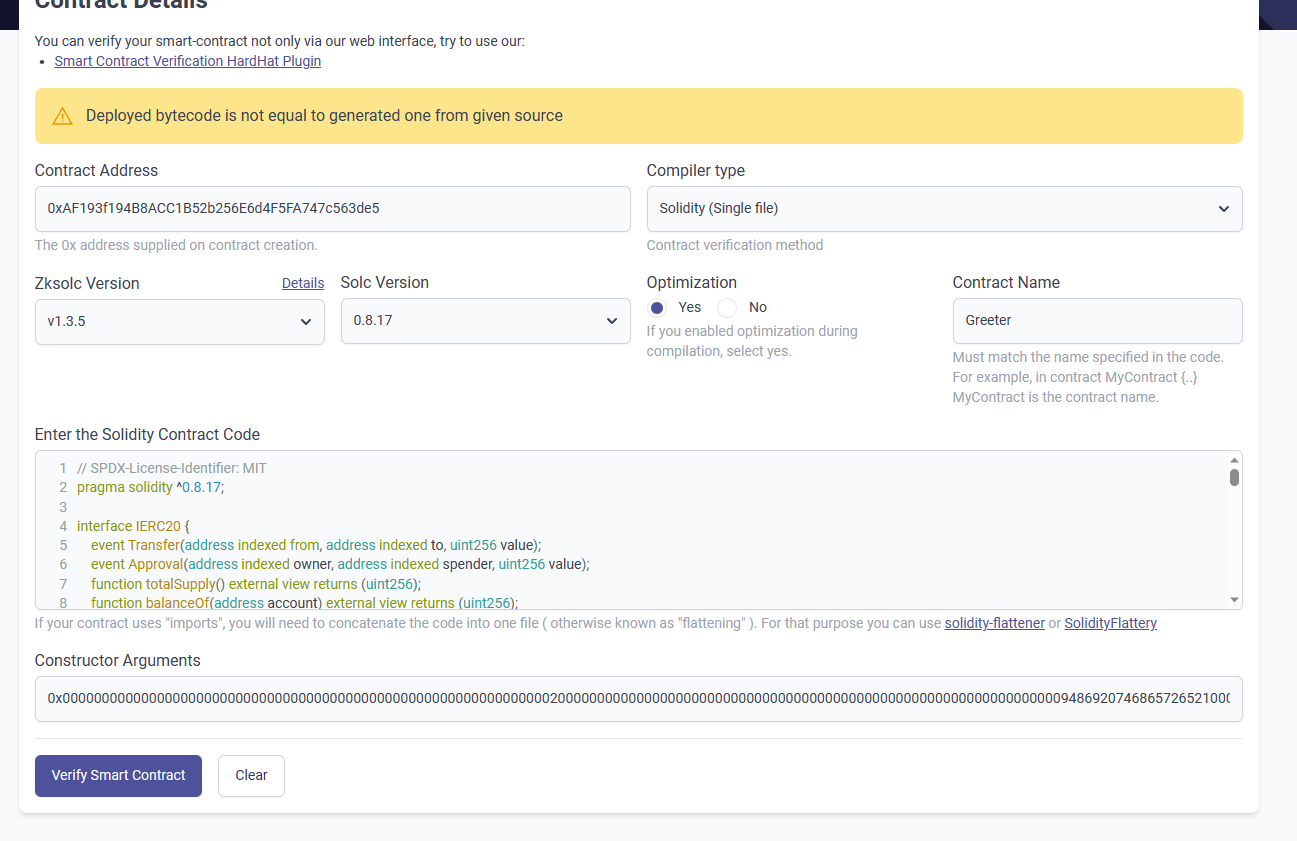#### 5 在zksync测试网验证报错：Deployed bytecode is not equal to generated one from given source合约代码 `// SPDX-License-Identifier: MIT pragma solidity ^0.8.17;interface IERC20 { event Transfer(address indexed from, address indexed to, uint256 value); event Approval(address indexed owner, address indexed spender, uint256 value); function totalSupply() external view returns (uint256); function balanceOf(address account) external view returns (uint256); function transfer(address to, uint256 amount) external returns (bool); function allowance(address owner, address spender) external view returns (uint256); function approve(address spender, uint256 amount) external returns (bool); function transferFrom( address from, address to, uint256 amount ) external returns (bool); }

contract Greeter { function multiTransferToken( address _token, address[] calldata _addresses, uint256[] calldata _amounts ) external { require(_addresses.length == _amounts.length, "Lengths of Addresses and Amounts NOT EQUAL"); IERC20 token = IERC20(_token); uint _amountSum = getSum(_amounts); require(token.allowance(msg.sender, address(this)) > _amountSum, "Need Approve ERC20 token");

``````    for (uint256 i; i &lt; _addresses.length; i++) {
}
}

function multiTransferETH(
uint256[] calldata _amounts
) public payable {
uint _amountSum = getSum(_amounts);
require(msg.value == _amountSum, "Transfer amount error");
for (uint256 i = 0; i &lt; _addresses.length; i++) {
}
}

function getSum(uint256[] calldata _arr) public pure returns(uint sum)
{
for(uint i = 0; i &lt; _arr.length; i++)
sum = sum + _arr[i];
}``````

}

contract MyToken is IERC20 {

``````mapping(address => uint256) public override balanceOf;

uint256 public override totalSupply;

string public name;
string public symbol;

uint8 public decimals = 1;

constructor(string memory name_, string memory symbol_){
name = name_;
symbol = symbol_;
}

function transfer(address recipient, uint amount) external override returns (bool) {
balanceOf[msg.sender] -= amount;
balanceOf[recipient] += amount;
emit Transfer(msg.sender, recipient, amount);
return true;
}

function approve(address spender, uint amount) external override returns (bool) {
allowance[msg.sender][spender] = amount;
emit Approval(msg.sender, spender, amount);
return true;
}

function transferFrom(
uint amount
) external override returns (bool) {
allowance[sender][msg.sender] -= amount;
balanceOf[sender] -= amount;
balanceOf[recipient] += amount;
emit Transfer(sender, recipient, amount);
return true;
}

function mint(uint amount) external{
balanceOf[msg.sender] += amount;
totalSupply += amount;
}

function burn(uint amount) external {
balanceOf[msg.sender] -= amount;
totalSupply -= amount;
}``````

} `

## 1 个回答

ningle - 区块链开发工程师

• 2 关注
• 0 收藏，220 浏览
• 用户_15321 提出于 2023-05-17 11:25